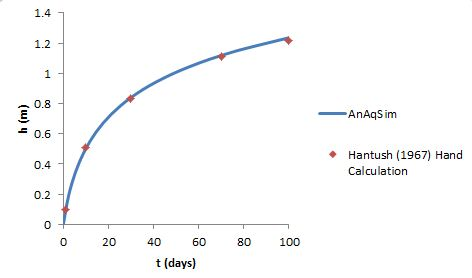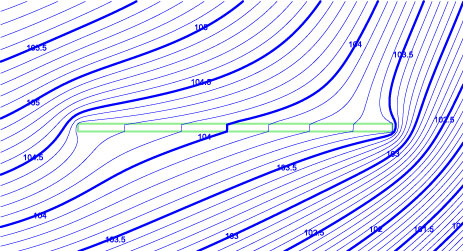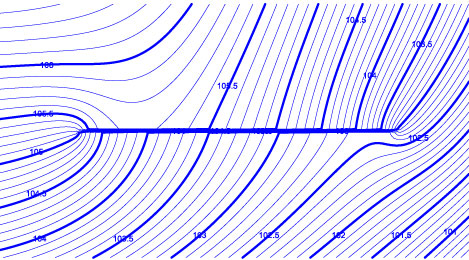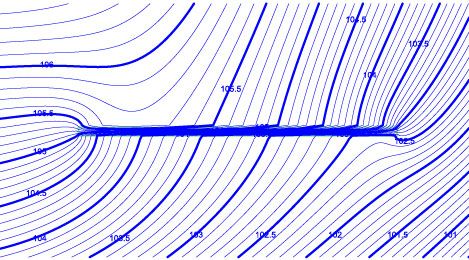## Checks of AnAqSim

To save any of the associated files, right-click on the file link and select “Save link as…”.

##### Check 1: Uniform flow in a confined aquifer.

Checks confined domain, constant head line boundary, normal flux line boundary, pathlines. In this single-domain model, the head declines from 30 at x=0 to 10 at x=1000, for a hydraulic gradient of 0.02.  The confined aquifer is 10 units thick and has a isotropic uniform K = 3 and porosity of 0.20.  The model uses head-specified line boundaries and normal flux-specified boundaries to create the uniform flow.  The model solves in one iteration using an underrelaxation factor of 1.0, since all boundary conditions are linear.  Making a contour plot shows the uniform slope of the water table.  The specific discharge, in accord with Darcy’s Law, is 0.06 and the average linear velocity is 0.30, which can be confirmed by moving the cursor around and examining the output.  The single pathline goes 1000 ft, and the arrowhead information indicates this takes 1000/0.3 = 3333 time units, as it should.  The aquifer discharge (specific discharge times saturated thickness) is 0.6, as it should be.  Input file: check1.anaq

##### Check 2: Uniform flow in a confined aquifer with uniform recharge.

Checks confined domain, constant head line boundary, normal flux line boundary, uniform recharge, 3D pathlines.  This is the same as check1, with the addition of uniform flux into the top of the domain (recharge) at a rate of 0.0005.  Flow over the entire model is from left to right.  Over the 1000 unit distance from left to right, the uniform recharge adds an additional 0.50 to the domain discharge, which it does.  The domain discharge (specific discharge times saturated thickness) is about 0.35 at the left edge and about 0.85 at the right edge.  The one pathline starts at the left edge at the top of the domain.  By the time it reaches the right side, recharge has added 0.50 units of discharge above the pathline, compared to 0.35 below it for a total domain discharge of 0.85.  At the right side, the fractional depth of the pathline is 0.5/0.85 = 0.59, as is should be, which places the pathline at an elevation of about 4.1 in the domain that goes from 0 to 10 elevation.  Input file: check2.anaq

##### Check 3: Uniform flow in a confined aquifer with uniform leakage from below.

Checks confined domain, constant head line boundary, normal flux line boundary, uniform leakage from below, 3D pathlines. This is the same as check 2, but with the leakage coming from below and the pathline starting at the bottom of the aquifer on the left.  The discharges are the same as in check2, but this time the pathline rises to a fractional depth of 0.41 at the right side (elevation 5.9), as it should.  Input file: check3.anaq

##### Check 4: Uniform flow in an unconfined aquifer with uniform recharge.

Checks unconfined domain, constant head line boundary, normal flux line boundary, uniform recharge, 3D pathlines. This is the same as check2, but with an unconfined aquifer instead of a confined one.  The base of the aquifer is at 0.  At the left edge of the model, the domain discharge is about 0.93.  The recharge adds 0.50 as the pathline crosses the model from left to right, so the total discharge at the right side is 1.43.  The fractional discharge above the pathline at the right side of the model is 0.5/1.43 = 0.35, which puts it at about elevation 6.5.  Checking the specific discharge, average linear velocity and domain discharge anywhere in the model confirms consistency with Darcy’s Law.  Input file: check4.anaq

##### Check 7: Uniform flow in an unconfined aquifer with uniform recharge and two levels.

Checks unconfined and confined domains, constant head line boundary, normal flux line boundary, spatially-variable area sinks for leakage, 3D pathlines traced across multiple levels. This is the same as check 4, but with two model layers: an unconfined aquifer over a confined one.  The base of the unconfined domain is at 5 and the confined domain goes from 0 to 5 elevation.  A spatially-variable area sink simulates vertical leakage between the two levels.  At the left edge of the model, the unconfined domain discharge is about 0.78 and the confined domain discharge is about 0.16.  The recharge adds 0.50 as the pathline crosses the model from left to right, so the total discharge at the right side is 0.78 + 0.16 + 0.50 = 1.44 (0.80 in the upper + 0.64 in the lower at the right side).  The pathline that started at the top of the upper level at the left side stays in the in the upper domain and its fractional depth in level 1 at the right side of the model is 0.5/0.80 = 0.62, which puts it at about elevation 6.95 = 5 + (1.0-.62) * (10 – 5).  A second pathline starts at the left edge of the model in the middle of the upper level (elevation 17.5).  At the left edge, 0.78/2 = 0.39 is the discharge above the pathline.  When this pathline reaches the right edge of the model, there is 0.5 + 0.38 = 0.88 discharge above the pathline, which includes all of the upper level discharge (0.80) plus 0.08 in the lower level.  The fractional depth of this pathline at the right side of the model is 0.08/0.64 =0.13 in the lower level level (elevation = 5 * (1.0 –.13) = 4.3).  Checking the extraction along a line from 0, 500 to 1000, 500 shows a good approximation of the spatially-variable distribution of vertical leakage in both levels.  Input file: check7.anaq

##### Check 8: Uniform flow in a two-level confined aquifer system with a circular opening connecting the two levels, like example 31.1 on pages 369-370 of Strack (1989).

Checks confined domains, constant head line boundary, normal flux line boundary, inter-domain boundaries with two levels transitioning to a single level. This is like Strack’s example 31.1, with uniform flow in the upper level far-field, and no flow in the lower level far-field far-field (far-field approximated by distant line boundary conditions).  The layer thickness is H = 10.  Head contour plots in both levels compare well with Figure 5.8 of Strack (1989).  Input file: check8.anaq

##### Check 9: Transient simulation of theTheis (1935) solution.

Checks confined domains, spatially-variable area sinks for simulating storage changes. This is a single confined domain with K = 3, thickness of 10, T = 30, S = 0.001.  One well pumps at a constant discharge.  Initial heads are uniform at 100.  The simulation is transient with one 3-unit time period, 15 time steps, and a time step multiplier of 1.2.  Simulated hydrographs are generated at r = 2, 20, and 200.  These are compared in the following figure to hydrographs computed from the Theis solution as programmed in the TWODAN software (listed as “Obs…” in the graph).  Checking the transient extraction along a line through the well indicates good approximation of the governing equation with this model.  A plot of transient heads through the well is shown in the 2nd figure below.   Input file: check9.anaq.  Tab-delimited text file with the Theis solution data: check9_TheisData.txt.##### Check 10: Transient simulation of the Hantush (1967) analytic solution for mounding under a rectangular recharge area.

Checks unconfined domains, spatially-variable area sinks for simulating storage changes and spatially-variable recharge, inter-domain boundary. This is an unconfined aquifer that is 6 m of saturated thickness.  The rectangular recharge area starts adding recharge at a steady rate of 0.05 m/day at time zero.  The recharge area is 60 m x 20 m.  The aquifer horizontal K = 2 m/day and the specific yield is Sy = 0.12.  The AnAqSim simulation starts with heads = 0 (aquifer base = –6.0 m), it runs for one 100 day time period with 10 time steps and a time step multiplier of 1.3.  A hydrograph is generated at one of the corners of the recharge area, and this is compared to hand calculations of mounding at this point, computed with Equation 14 in the Hantush (1967) paper (Water Resources Research, 3(1), p. 227).  The results are as close as possible given the interpolation from tables required for the hand calculation.  The AnAqSim-generated head contours at t = 100 days is shown below with the recharge area outlined in green.  Input file: check10.anaq.##### Check 11: Comparison of complex multi-level AnAqSim model and equivalent MODFLOW 2005 model.

Checks confined, unconfined domains, transient and steady flow, spatially-variable area sinks for simulating vertical leakage and storage changes, anisotropy, wells, constant head line boundaries, normal fllux line boundaries, and inter-domain line boundaries.  Several files may be downloaded to examine and run this check:

• Model description and plots comparing results of steady and transient models: CompareModels.pdf
• AnAqSim input files and the starting head file for the transient runs: AnAqSimFilesCheckMF.zip
• MODFLOW input files and ModelMuse input file that created the MODFLOW input: MFCheckTR.zip

This check demonstrates that AnAqSim and MODFLOW produce similar results for a complex model that implements most of AnAqSim’s capabilities.  The comparisons are similar for both steady state and transient simulations.

##### Check 12: Check of AnAqSim drain line boundary.

Checks drain line boundary, interdomain boundary.  This compares two models with uniform recharge within a circular constant head boundary.  The two models are identical, but one has a drain constructed with a line drain element and in the other, the drain is represented as a separate domain with finite width and the same equivalent conductivity as the line drain.  The plots below are plots of heads near the drain in the two models and show nearly identical patterns.  The files for the two models are DrainCheck1.anaq and DrainCheck2.anaq.##### Check 13: Check of AnAqSim leaky barrier line boundary.

Checks leaky barrier line boundary, interdomain boundary. This compares two models with uniform recharge within a circular constant head boundary.  The two models are identical, but one has a leaky barrier constructed with a leaky barrier line boundary and in the other, the leaky barrier is represented as a separate domain with finite width and the same equivalent resistance as the leaky barrier line boundary.  The plots below are plots of heads near the barrier in the two models and show nearly identical patterns.  The files for the two models are LeakyBarrierCheck1.anaq and LeakyBarrierCheck2.anaq.##### Check 14: Uniform flow in a confined/unconfined aquifer with uniform recharge.

Checks confined/unconfined domain, constant head line boundary, normal flux line boundary, uniform recharge, 3D pathlines.  This is the same as check2, but with a combined confined/unconfined domain.  The domain goes from elevation 0 to 10.  Head at the left is fixed at 15 (confined) and head at the right is fixed at 2 (unconfined).  Flow over the entire model is from left to right.  Over the 1000 unit distance from left to right, the uniform recharge adds an additional 0.50 to the domain discharge, which it does.  The domain discharge (specific discharge times saturated thickness) is about 0.044 at the left edge and about 0.544 at the right edge.  At the transition from confined to unconfined (h=10), the head, domain discharge, specific discharge, pathline elevation, and saturated thickness are all continuous as they should be.  Within both the confined and unconfined portions of the model these variables are all properly related.  Input file: check14.anaq

##### Check 15: Uniform flow in a two-layer confined/unconfined aquifer with uniform recharge.

Checks confined/unconfined domain, spatially-variable area sinks for vertical leakage, constant head line boundary, normal flux line boundary, uniform recharge, 3D pathlines, minimum saturated thickness where head drops to base of unconfined domain.  This is the same as check14, but with two layers, both of which are combined confined/unconfined domains.  The upper domain (level 1) spans elevation 5-10 and the lower domain (level 2) spans elevation 0-5.  The vertical conductivity is high enough that there are very small head differences between the two levels, so the solution should be nearly identical to check 14, which it is.  Heads in level 1 fall below the base (5) at the right side of the model, where the minimum saturated thickness (0.0001 * 5) is invoked; discharges in this zone are very small.  The graph below shows aquifer discharge (Qx) as a function of x in each layer and the sum of the two.  The sum is nearly identical to a similar plot for check 14, as expected.  Input file: check15.anaq.##### Check 16: Head-dependent normal flux (3rd type) boundary

Checks head-dependent normal flux (3rd type) line boundary, interdomain boundary, confined and unconfined domains.  This compares two single-level models that both have a confined domain that is 1000 x 1000 units in the x and y directions, specified normal flux = 0 (no-flow) boundaries at y = 500 and y =-500, and a constant head boundary h = 12.0 at x = 500.

• One model uses the head-dependent normal flux boundary at x = -500 with conductance C/b = 0.001 and h* = 15.0, (Check3rdType.anaq).
• The other model doesn’t have the 3rd type boundary, but instead has an equivalent finite width 2nd domain that extends from -600 < x < -500. The transmissivity (T) of this 2nd domain is 1/10th the T of the other domain. A head-specified boundary with h = 15.0 is at x = -600. The conductance of this 2nd domain is equivalent to the boundary conductance C/b = 0.1/100 = 0.001 in the other model (Check3rdType_alternate.anaq).

Both models result in h=13.50 and domain discharge Qx = -T (dh/dx) = 0.01500 at x=-500. See the contour plots compared below.  Changing the model so that it is unconfined yields similar good comparisons between the model with the 3rd type boundary and the model with an equivalent finite 2nd domain (Check3rdType_unconfined.anaq, Check3rdType_unconfined_alternate.anaq).

##### Check 17: Check of vertical leakage over polygon tool

Checks the Vertical Leakage over Polygon Area tool.  This model has two levels, one domain in each level.  The domains are circular with radius R = 100 [L].  The specified top flux (recharge) = 0.01 [L^2/T].  So the total vertical flux into the top of the model = pi * R^2 = 314.159 [L^3/T].  There is a well at the center of the circle pumping at a rate of 300 [L^3/T].  The upper level has a specified head boundary around its perimeter, and the lower level has a no-flow boundary around its perimeter.  Using the Vertical Leakage over Polygon Area tool for the area of the whole model gives 312.3 entering the top of the model, and 299.4 entering the lower level of the model.  These are close to the ideal values, and differences are due to the numerical integration scheme employed by the tool; rates are evaluated at points, multiplied by representative areas, and summed. (checkPolygonLeakage.anaq)

##### Check 18: Multi-layer pumping test comparison with MLU

Checks multi-level flow, partially-penetrating multi-level well, transient flow, spatially-variable area sinks (SVAS).  This is a model of a pumping test in a thick, stratified aquifer in northern California.  It has been modified slightly (no unconfined layer at top, initial heads are everywhere = 0) so that a direct comparison could be made between AnAqSim and MLU, which is an analytic multi-level model that uses Laplace transforms and analytic elements (LT-AEM) to get exact distributions of vertical leakage and storage fluxes, without finite time steps.  This contrasts AnAqSim, which approximates the leakage and storage distributions using SVAS, and uses finite difference time steps.  AnAqSim’s SVAS approach adds many important capabiliites such as unconfined and fresh/salt interface domains and a wide range of line boundary conditions not available with the LT-AEM approach in MLU .The models have 9 layers that are all confined and isotropic in the plane of the layering, as shown at left.  The vertical hydraulic conductivity of each layer equals 1/5th the horizontal conductivity.  The pumping well spans three of the 9 levels including the very transmissive 5th layer.  Three shallow observation wells shown in the section were monitored in addition to the pumping well.  The AnAqSim model uses a large number of time steps to minimize differences due to finite difference time stepping.

The model-simulated drawdowns in both the pumping well and in the three observation wells are essentially identical as the following plots show.  AnAqSim simulation results are the lines and MLU simulation results are dots. (checkPumpingTestMLU.anaq)##### Check 19: 3D pathline tracing comparison with Modflow

Checks 3D pathline tracing.  This is a model of flow in the X direction in an unconfined, steady, single layer model with uniform recharge at the top.  There are two domains, both with horizontal K = 50 and a uniform recharge of 0.004566.  The left side (x<2500) has a base elevation of 0 and the right side domain has a base elevation of -5, creating a step down in the base.  The head goes from 95 at x=0 to 85 at x=5000.  A single pathline is traced downstream from the water table at x=600.  The plot below compares a vertical profile of the pathline traces in the AnAqSim model and an equivalent MODFLOW/Modpath model; they are essentially identical.  Both show a vertical jump in the pathline where the aquifer base drops, as required to maintain the same fractions of flow above and below the pathline. Thanks to folks at McLane Environmental for sharing this comparison.  The AnAqSim model file is Check19.anaq and the MODFLOW/Modpath input files are in this zip file: PathlineStepModflowModpathFlies.zip.